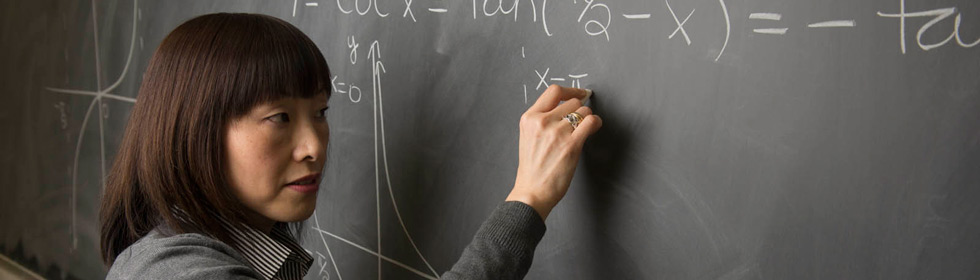Department of Mathematics & StatisticsThe Department offers a graduate degree of Master of Science in Mathematics with specializations in:

• Mathematics
• Statistics and Operations Research
• Computational and Applied Mathematics
• Post-secondary Mathematics Education

You can view a list of some recent theses and special projects written by our graduate students here.

### Program of Study

Students are assigned an academic advisor to plan an appropriate program of study. Requirements for each specialization appear in more detail in the Graduate Catalog.

### Pure Mathematics

This specialization focuses on the theory underlying modern mathematics. It prepares students for further advanced study in mathematics, community college teaching, and fields where a solid grounding in theoretical mathematics is required.

• Required Courses
• Math 421 (Linear Algebra II)
• Math 430 (A Geometric Introduction to Topology) or 437 (Differential Geometry)
• Math 450 (Real Analysis I)
• Math 451 (Complex Analysis) or Math 552 (Theory of Ordinary Differential Equations)
• Math 555 (Functional Analysis) or Math 565 (Advanced Numerical Analysis)
• Math 520 (Topics in Algebra) or Math 545 (Real Analysis II)
• Electives (9 hours)
• Thesis, special project, or exam (with additional 500 level course)

### Computational and Applied Mathematics

This specialization may be of interest to students with undergraduate majors in mathematics, computer science, engineering, and physics, who need to use computers to solve industrial and scientific problems. Graduates choosing this option receive training in the formulation of mathematical solutions to industrial or scientific problems and the development and implementation of algorithms for the solution of such problems.

• Required Courses
• Math 421 (Linear Algebra II)
• Math 450 (Real Analysis I)
• Math 464 (Partial Differential Equations)
• Either Math 465 (Numerical Analysis) or Math 466 (Numerical Linear Algebra with Applications)
• Either Math Math 555 (Functional Analysis) or Math 565 (Advanded Numerical Analysis)
• Either Math 451 (Complex Analysis) or Math 552 (Theory of Ordinary Differential Equations)
• Electives (9 hours; Math 550, 567, Stat 575, and OR 587ab are recommended)
• Thesis, special project, or exam (with additional 500 level course)

### Statistics and Operations Research

Our graduate program in Statistics and Operations Research gives students the foundation to apply mathematical and statistical methods in industry, government, and scientific research. Graduates of our program have positions in business, academia, and government.

• Required Courses
• Stat 480a and 480b (Mathematical Statistics)
• One of: OR 440 (Deterministic Models), OR 587a (Mathematical Programing), Math 423 (Combinatorics and Graph Theory)
• One of: OR 441 (Stochastic Models), OR 442 (Simulation), OR 585 (Advanced Simulation Modeling)
• One of the following two semester sequences Stat 581/582, Stat 584/588, Stat 579/589, OR 587a/587b, OR 585/586 (see note below)
• Electives (9 hours)
• Thesis, special project, or exam (with additional 500 level course)

A student taking the OR 587a/b sequence will be required to take either OR 440 or Math 423.  A student taking the OR 585/586 sequence will be required to take either OR 441 or OR 442.

### Post Secondary Mathematics Education

The post-secondary math education specialization contains both mathematics theory and pedagogy. Graduates will be well positioned to obtain a teaching position at a community college or teach dual-credit courses from many universities.

• Three courses from the following
• Math 420, 421, 423, 430, 435, 437, 450, 451, 464, 465, 466, OR 440, Stat 478, 480a, 480b, 481, 482
• Two content and pedagogy courses
• Math 531 (Algebraic Content, Pedagogy, and Connections)
• Math 532 (Geometric Content, Pedagogy, and Connections)
• Math 533 (Discrete Mathematics Content, Pedagogy, and Connections)
• Math 534 (Calculus Content, Pedagogy, and Connections)
• Stat 535 (Probability and Data Analysis Content, Pedagogy, and Connections)
• Two 500-level MATH/STAT/OR electives other than those above
• Two courses from the following
• ADED 522, ADED 523, EPFR 501, EPFR 515, CI 510, CI 563, IT 540
• Thesis, special project, or exam (with additional 500 level course)

### Forms

Contact Dr. Junvie Pailden (jpailde@siue.edu), Graduate Program Director of the Department for additional information.